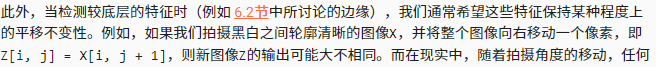# 汇聚层

pooling是否应该统一翻译成“汇聚”？

2 Likes
1. 设卷积层大小是m x n，卷积层里面每个元素参数是1 / (m*n)，这样就是一个平均汇聚层作为卷积层的实现
2. c * floor((h - ph + sh) / sh) * floor((w -pw + sw) / sw)
3. 不用softmax是因为softmax也有自己参数，这样模型的参数会非常多？？（不确定
抛砖引玉，希望大家有机会和大家讨论
1 Like
``````def corr_2d(X,K):
h = K.shape
w = K.shape
Y = torch.zeros((X.shape-h+1,X.shape-w+1))
for i in range(Y.shape):
for j in range(Y.shape):
Y[i,j] = (X[i:i+h,j:j+h] * K).sum()
return Y
def pool2d(X,pool_size,pool_mode):
h,w = pool_size
Y = torch.zeros((X.shape-h+1,X.shape-w+1))
for i in range(Y.shape):
for j in range(Y.shape):
if pool_mode == 'max':
Y[i,j] = X[i:i+h,j:j+w].max()
elif pool_mode == 'mean':
Y[i,j] = X[i:i+h,j:j+w].mean()
return Y

#1.将平均汇聚层作为卷积层的特殊情况实现,令输入张量为X[[1,2,3],[4,5,6],[7,8,9]]，卷积核为[[1/4,1/4],[1/4,1/4]]
X = torch.Tensor([[1,2,3],[4,5,6],[7,8,9]])
K = torch.Tensor([[1/4,1/4],[1/4,1/4]])
conv = corr_2d(X,K)
pool_mean = pool2d(X,(2,2),'mean')
conv,pool_mean
#2.将最大汇聚层作为卷积层的特殊情况实现,令输入张量为X[[1,2,3],[4,5,6],[7,8,9]]，卷积核为[[0,0],[0,1]]
X = torch.Tensor([[1,2,3],[4,5,6],[7,8,9]])
K = torch.Tensor([[0,0],[0,1]])
conv = corr_2d(X,K)
ph,pw = (2,2)
pool_mean = pool2d(X,(ph,pw),'max')
conv,pool_mean
#3. pool_mean * pool_mean * ph * pw * c
``````

4，5题都是个人理解，如果说得不对希望能够指正
4. 个人认为最大汇聚层适合提取那些像素值方差比较大的区域，比如把一个物体从它的背景提取出来，而平均汇聚层适合提取那些像素值方差比较小的区域，比如物体本身，这样更有效的保存了区域的有效特征。希望有人解答。。。
5. 我觉得需要，最小汇聚层能够提取那些像素值比较小的特征，比如黑色是0，白色是1的话，就能够把一个区域的黑色给提取出来。已知函数，这个还真不知道。。
6. Softmax的方法计算复杂度肯定是高于max和mean的

In reality, objects hardly ever occur exactly at the same place.

• 这句话的翻译有些奇怪，原义是否是：“而在现实中，物体几乎不可能准确地出现在相同的位置。”

1 LikeQ1: 你能将平均汇聚层作为卷积层的特殊情况实现吗？
Q2: 你能将最大汇聚层作为卷积层的特殊情况实现吗？

``````def pool2d_by_conv2d(X, pool_size, mode='max'):
K = torch.ones(pool_size)
h, w = K.shape
if mode == 'avg':
K.fill_(1 / (h * w))
Y = torch.zeros((X.shape - h + 1, X.shape - w + 1))
for i in range(Y.shape):
for j in range(Y.shape):
if mode == 'max':
Y[i, j] = (X[i:i + h, j:j + w] * K).max()
elif mode == 'avg':
Y[i, j] = (X[i:i + h, j:j + w] * K).sum()
return Y

X = torch.tensor([[0.0, 1.0, 2.0], [3.0, 4.0, 5.0], [6.0, 7.0, 8.0]])
print(pool2d(X, (2, 2)))
print(pool2d(X, (2, 2), 'avg'))

print(pool2d_by_conv2d(X, (2, 2)))
print(pool2d_by_conv2d(X, (2, 2), 'avg'))
``````

Q3: 假设汇聚层的输入大小为c×h×w，则汇聚窗口的形状为p_h×p_w，填充为(p_h,p_w)，步幅为(s_h,s_w)。这个汇聚层的计算成本是多少？
A3: 计算成本：c×(⌊(ℎ+𝑠_ℎ)/𝑠_ℎ⌋×⌊(𝑤+𝑠_𝑤)/𝑠_𝑤⌋)×p_h×p_w

Q4: 为什么最大汇聚层和平均汇聚层的工作方式不同？
A4: 没看懂题目啥意思…

Q5: 我们是否需要最小汇聚层？可以用已知函数替换它吗？
A5:

Q6: 除了平均汇聚层和最大汇聚层，是否有其它函数可以考虑（提示：回想一下softmax）？为什么它不流行？
A6: 将softmax引入汇聚层也可以，但softmax并不改变各个pixel之间的大小关系，在某种程度上与最大汇聚层的效果类似（最终选出的pixel和最大汇聚层选出的pixel一样，只是值不同），但增加了不少的计算量（指数运算），最终效果却不一定比最大汇聚层好。

1 Like
1. Q3中根据
6.3. 填充和步幅 — 动手学深度学习 2.0.0 documentation
中6.3.1公式，我觉得应该是
c×(⌊(ℎ-p_h+2p_h+𝑠_ℎ)/𝑠_ℎ⌋×⌊(𝑤-p_w+2p_w+𝑠_𝑤)/𝑠_𝑤⌋)×p_h×p_w
类似卷积计算量计算思路：把汇聚窗口当作卷积核，先根据6.3.1公式计算输出大小，即：
⌊(ℎ-p_h+2p_h+𝑠_ℎ)/𝑠_ℎ⌋×⌊(𝑤-p_w+2p_w+𝑠_𝑤)/𝑠_𝑤⌋
然后乘上c（通道数）
核大小p_h×p_w（汇聚窗口的形状为p_h×p_w）
另外，我觉得题目不太好的地方是将汇聚窗口的形状设置为和填充一样，公式可以化简，导致大家可能感受不到本质，既然是公式运算，不是同一个东西尽量使用不同的字符我觉得更具有普遍性
2. Q4: 为什么最大汇聚层和平均汇聚层的工作方式不同？
答：根据李沐老师视频里面展示的效果，最大汇聚层是强化突出作用，平均汇聚层是平滑过渡作用，所以一个是将局部最大值输出，一个是将局部均值输出
如果有任何不对的地方，欢迎指正